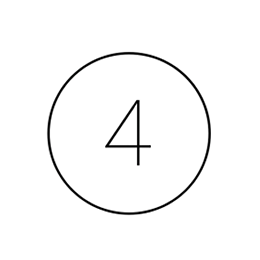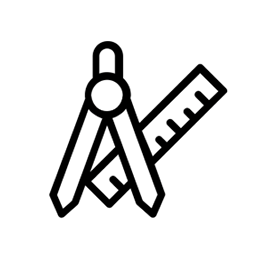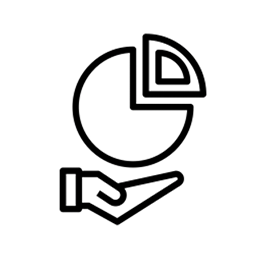# Mathematics

## Our Vision

Maths is a vital component of everyday life and helps us to understand and function in the world around us. As such our students will be taught to develop fluency, to reason mathematically, and to problem solve. We strongly believe that maths is best learned with the time to really delve into topics and allow students to work firstly on fluency and then on application. As such we deliver large chunks of content at a time, and revisit these regularly so that students can make links from prior work to new ideas.

At Torpoint it is our aim that all students leave:

• with a greater ability to problem solve, reason, explain, and justify
• knowing that they are resilient when faced with problems
• being numerate and can function well as members of society
• being confident and competent with mathematical techniques

## KS3

Each year they will cover different elements and then in subsequent years they will review prior work before building on these foundations of knowledge. We firmly believe that all students should be introduced to as many different concepts and techniques as possible which is why we deliver both Foundation and Higher tier content to all students for as long as we feel they can manage it.

## KS4

At KS4 we follow the specifications for Edexcel. Students study all of the concepts below and have plenty of time to practise key skills that underpin the subject discipline and complete exam style questions.

## KS5

At KS5 we offer A level mathematics and this is the chance for students to really hone their expertise. It is a demanding but hugely interesting A level.

## Key ConceptsNumberAlgebraGeometryRatio and ProportionStatistics and probability

## KS3 Mathematics

### Curriculum Breakdown

We will continually adapt and re-order our content so that it maximises progress for each cohort depending on where strengths and weaknesses lie, how often we need to revisit topics, or where we can easily build on secure foundations of knowledge. The following table is a guide, please be aware this may be adapted.

## Year 7

• Understand and be able to apply their knowledge of integers and decimals including all four operations
• Understand and be able to manipulate algebra according to correct rules
• Understand and be able to use coordinates in all four quadrants
• Understand and be able to use the language and reasoning of lines, angles and shapes
• Know and understand how to collect data including planning to avoid bias and sampling techniques
• Understand and be able to use similarity and congruence when looking at shapes
• Understand and be able to apply their knowledge of different patterns and sequences including using algebra to describe them
• Understand and be able to apply their knowledge of fractions including the four operations

## Year 8

• Know formal constructions and can use these when working with loci
• Be able to read and interpret scales, and understand how to convert units (metric/metric)
• Understand and use a variety of charts and diagrams to display given or collected data
• Understand pie charts and can use or construct them accurately
• Know and be able to use different types of numbers
• Know and be able to use all properties of quadrilaterals
• Know the correct language and meaning of 2D and 3D shapes and their properties and can work with them effectively
• Be confident with the links between fractions, decimals and percentages and can use these in context
• Be able to apply their knowledge of percentages to different situations
• Know how to manipulate linear equations and inequalities in order to find solutions understand and can use formulae for areas and perimeters of 2D shapes

## Year 9

• Understand and be able to manipulate algebra including powers and brackets
• Understand and be able to apply knowledge of ratio, scale and proportion
• Understand and be able to construct, read and interpret real life graphs
• Know and be able to work effectively with straight line graphs including the use of y=mx+c
• Know and be able to use formulae effectively including those used in other subjects
• Understand all four transformations and can do and describe each of them
• Know all of the averages and measures of spread and can apply this knowledge to different sets of data
• Understand and can use both Pythagoras theorem and basic trigonometry: SOHCAHTOA
• Understand and can use standard form and index notation including manipulation
• Understand and can manipulate surds
• Understand and can apply each of the advanced trigonometric rules: sine rule and cosine rule, and know how to use trigonometry to find the area of a triangle (H only)

## KS4 Mathematics

### Curriculum Breakdown

• Understand how to read and construct timetables and distance time graphs as well as using these with confidence
• Understand and can work effectively with compound measures
• Know and can use correctly formulae for surface areas and volumes of 3D shapes
• understand the language of, and can work effectively with probability
• Understand and can work effectively with angles in polygons
• Know and be able to use all properties of parallel lines
• Know how to construct, use and interpret scatter graphs and discuss correlation
• Recognise and can sketch different functions including quadratics and can use these sketches to find approximate solutions
• Be able to apply knowledge of quadratics algebraically (H only)
• Understand and can use vocabulary and notation associated with vectors
• Be able to work with vectors geometrically (H only)
• Know and be able to use vocabulary and formulae associated with circles
• Know and can recognise and use each of the circle theorems, and have considered their proofs (H only)
• Understand how to manipulate and solve simultaneous equations both algebraically and graphically, including non-linear situations
• Recognise and can sketch different functions including trigonometric graphs and can use these to find solutions within a given range (H only)
• Understand function notation and transformations of functions (H only)